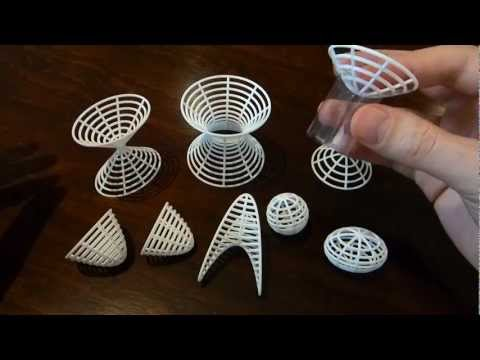DIGITAL PREVIEW
Not a Photo

With transparent film used to support the hyperboloid of two sheets.DIGITAL PREVIEW
Not a Photo

# Calculus surfaces (small)

\$72.58
3D printed in white nylon plastic with a matte finish and slight grainy feel.
QTY

You must be logged in and verified to contact the designer.
##### Product Description

This is a collection of common example surfaces from classes in multivariable calculus. Much larger versions of these surfaces are available here.

Contour lines, together with 8 radial curves make up the surfaces. All surfaces are plotted in such a way to show values of z in [-2,2]. The hyperbolic paraboloid is further cut along a cylinder of radius sqrt(2). The equations of the surfaces are:

• Elliptical cone: z = +- sqrt(2x^2 + y^2)
• Hyperboloid of one sheet: z = +- sqrt(x^2 + y^2 - 1)
• Hyperboloid of two sheets: z = +- sqrt(x^2 + y^2 + 1)
• Circular paraboloid: z = x^2 + y^2
• Elliptical paraboloid: z = 2x^2 + y^2
• Hyperbolic paraboloid: z = x^2 - y^2
• Sphere: z = +- sqrt(1 - x^2 - y^2)
• Ellipsoid: z = +- sqrt(1 - (x^2)/2 - y^2)

Note that the hyperboloid of two sheets is not connected. In the photo here I have propped them apart using a piece of overhead projector transparency sheet, rolled into a tube and secured with sticky tape.##### Details
What's in the box:
Calculus surfaces (small)Dimensions:
11.36 x 13.15 x 5.26 cm
Switch to inches
4.47 x 5.18 x 2.07 inches
Switch to cm
Success Rate:
First To try.
What's this?
Rating:
Mature audiences only.